### IMO Shortlist 1985 problem 4

Kvaliteta:
Avg: 0.0
Težina:
Avg: 0.0
Each of the numbers in the set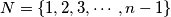$N = \{1, 2, 3, \cdots, n - 1\}$, where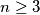$n \geq 3$, is colored with one of two colors, say red or black, so that:

(i)$i$ and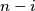$n - i$ always receive the same color, and

(ii) for some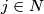$j \in N$, relatively prime to$n$,$i$ and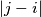$|j - i|$ receive the same color for all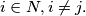$i \in N, i \neq j.$

Prove that all numbers in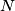$N$ must receive the same color.
Source: Međunarodna matematička olimpijada, shortlist 1985